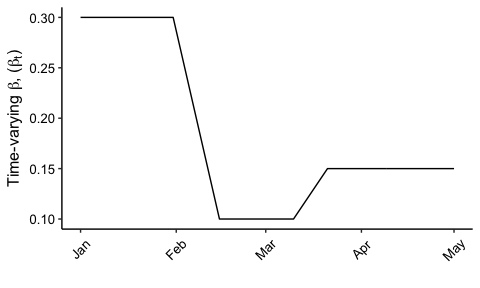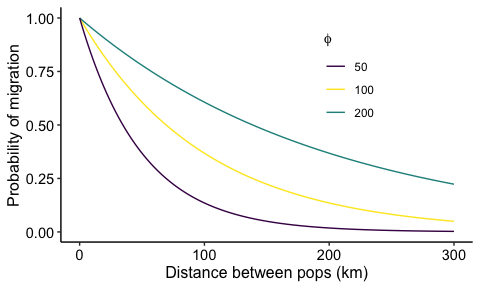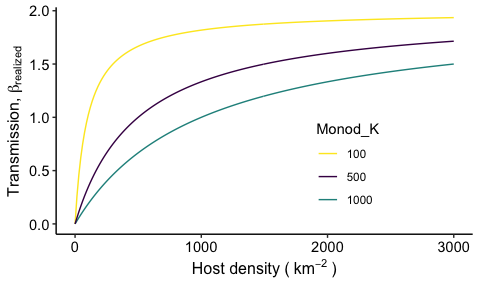# SPARSE-MOD Key Features

#### July 2022

library(tidyverse)
library(viridis)
library(lubridate)

## SPARSE-MOD: Overview and Key Features

SPARSE-MOD stands for SPAtial Resolution-SEnsitive Models of Outbreak Dynamics. Our goal with this R package is to offer a framework for simulating the dynamics of stochastic and spatially-explicit models of infectious disease. As we develop the package, our goal is to add more model structures and more user-control of the model dynamics. Our SPARSEMODr package offers several key features that should make it particularly relevant for pedogogical and practical use. See our COVID-19 model vignette and our SEIR model vignettefor detailed walk-throughs of how to run the model(s), to plot the output, and to simulate customized time-windows.

• Spatially explicit models that allow user-defined meta-populations1 characteristics and a customizable dispersal kernel (see below).

• Customizable process time-windows: The user controls how model parameters can vary over time, such as the transmission rate, or parameters that define the host migration processes. We have created $$\texttt{time_window}$$ objects that allow users to simulate, for example, time periods over which public health or conservation interventions are implemented that can affect the contact rates between hosts or the movement of hosts among populations.

• Demographic stochasticity2 is built-in using a tau-leaping algorithm3. This captures the random transmission processes that are important early in outbreaks and especially in small host populations.

• Stochastic transmission is also built-in, allowing daily fluctuations in the transmission rate, which can help account for dynamics like super-spreading or super-shedding.

• The transmission process can be simulated as frequency-dependent (i.e., contact rates are invariable to population density) or density-dependent (i.e., contact rates depend on population density). For density-dependent transmission, we allow the user to custom-define a (non-)linear relationship between local host density and the transmission rate (see below).

• Models are coded in C++ and take advantage of Rcpp for rapid simulation of stochastic model trajectories across many focal populations. We also demonstrate how independent realizations of the stochastic models can be run in parallel using the $$\texttt{future}$$ R package.

## In-depth…

### Time windows

One of the benefits of the SPARSEMODr design is that the user can specify how the values of certain model parameters might change over time. In this particular example, we show how the time-varying transmission rate, $$\beta_{t}$$ might change in a stepwise fashion due to ‘interventions’ and ‘release of interventions’. We assume that when a parameter value changes between two time windows, there is a linear change over the number of days in that window. In other words, the user specifies the value of the parameter acheived on the last day of the time window. Note, however, that the user is instead allowed to supply daily parameter values to avoid this linear-change assumption. Here we show an example of a pattern of time-varying transmission rate that a user might specify, and how the C++ code is interpreting these values on the back-end.

# Set up the dates of change. 5 time windows
n_windows = 5
# Window intervals
start_dates = c(mdy("1-1-20"),  mdy("2-1-20"),  mdy("2-16-20"), mdy("3-11-20"),  mdy("3-22-20"))
end_dates =   c(mdy("1-31-20"), mdy("2-15-20"), mdy("3-10-20"), mdy("3-21-20"), mdy("5-1-20"))

# Date sequence
date_seq = seq.Date(start_dates, end_dates[n_windows], by = "1 day")

# Time-varying beta
changing_beta = c(0.3,            0.1,            0.1,            0.15,            0.15)

#beta sequence
beta_seq = NULL

beta_seq[1:(yday(end_dates) - yday(start_dates) + 1)] =
changing_beta

for(i in 2:n_windows){

beta_temp_seq = NULL
beta_temp = NULL

if(changing_beta[i] != changing_beta[i-1]){

beta_diff = changing_beta[i-1] - changing_beta[i]
n_days = yday(end_dates[i]) - yday(start_dates[i]) + 1
beta_slope = - beta_diff / n_days

for(j in 1:n_days){
beta_temp_seq[j] = changing_beta[i-1] + beta_slope*j
}

}else{
n_days = yday(end_dates[i]) - yday(start_dates[i]) + 1
beta_temp_seq = rep(changing_beta[i], times = n_days)
}

beta_seq = c(beta_seq, beta_temp_seq)

}

beta_seq_df = data.frame(beta_seq, date_seq)
date_breaks = seq(range(date_seq),
range(date_seq),
by = "1 month")

ggplot(beta_seq_df) +
geom_path(aes(x = date_seq, y = beta_seq)) +
scale_x_date(breaks = date_breaks, date_labels = "%b") +
labs(x="", y=expression("Time-varying "*beta*", ("*beta[t]*")")) +
# THEME
theme_classic()+
theme(
axis.text = element_text(size = 10, color = "black"),
axis.title = element_text(size = 12, color = "black"),
axis.text.x = element_text(angle = 45, vjust = 0.5)
)### Dispersal kernel

As we discuss in the documentation (see $$\texttt{?SPARSEMODr::Movement}$$), we allow migration between populations in the meta-population to affect local and regional transmission dynamics. For now, migration is determined by a simple dispersal kernel, although we are working on adding more customizable gravity kernels. The user can control the shape of this kernel with the $$\texttt{dist_phi}$$ option, as follows: $p_{i,j} = \frac{1}{\text{exp}(d_{i,j} / \phi)},$ where $$p_{i,j}$$ is the probability of moving from population $$j$$ to population $$i$$ and $$d_{i,j}$$ is the euclidean distance between the two populations.

We can see how $$\phi$$ (i.e., $$\texttt{dist_phi}$$ in the model input) controls the probability below. In general, larger values of $$\texttt{dist_phi}$$ make it more likely for hosts to travel farther distances.### Transmission Types

As we describe in the documentation (e.g., see $$\texttt{?SPARSEMODr::model_interface}$$), we allow the user to implement frequency-dependent (FD) or density-dependent (DD) transmission in the SPARSEMODr models.

For FD transmission, we divide the user-specified value of transmission rate by the total number of hosts within a given sub-population. For example, in the classic SEIR model, where S, E, I, and R, are the numbers (i.e., integers) of susceptible, exposed, infectious, and recovered hosts, respectively, we would have the following expression describing mass-action transmission per sub-population, $$i$$: $\beta_i \frac{S_i}{N_i} I_i,$ where $$\beta_{i}$$ is the user-specified transmission rate for sub-population $$i$$, and $$N_{i}$$ is the total host population size for sub-population $$i$$. Therefore, with FD transmission, the effect of a single infectious host on the risk of infection is modulated by the fraction of the host population that is still susceptible, irrespective of the host population density (i.e., number of hosts per unit area) within that sub-population.

Alternatively, for DD transmission, the user can specify a (non-)linear Monod equation that describes the relationship between host population density and the transmission rate $$\beta$$ via the model’s (optional) parameter, $$\texttt{dd_trans_monod_k}$$. The Monod equation is: $\beta_{\text{realized}} = \beta_{\text{max}} \frac{\text{Dens}}{K + \text{Dens}},$ where $$\beta_{\text{max}}$$ is the maximum possible transmission rate across all densities, $$\texttt{Dens}$$ is the density of the focal host population (i.e., number of hosts per unit area), and $$K$$ is a constant that controls the effect of density on the transmission rate and is user-controlled by specifying $$\texttt{dd_trans_monod_k}$$. More specifically, $$K$$ is the half-velocity constant at which point $$\beta_{\text{realized}}/\beta_{\text{max}} = 0.5$$.

We can see how $$\texttt{dd_trans_monod_k}$$ controls the transmission rate below. In general, larger values of $$\texttt{dd_trans_monod_k}$$ mean that transmission rate is more strongly limited by population density.1. A set of distinct, focal populations that are connected by migration↩︎

2. The effects of probabilistic events that befall a population and that can affect epidemic trajectories.↩︎

3. Models are based off of differential equation models, but we use a tau-leaping algorithm - in the Gillespie family - to simulate the model one day at a time.↩︎